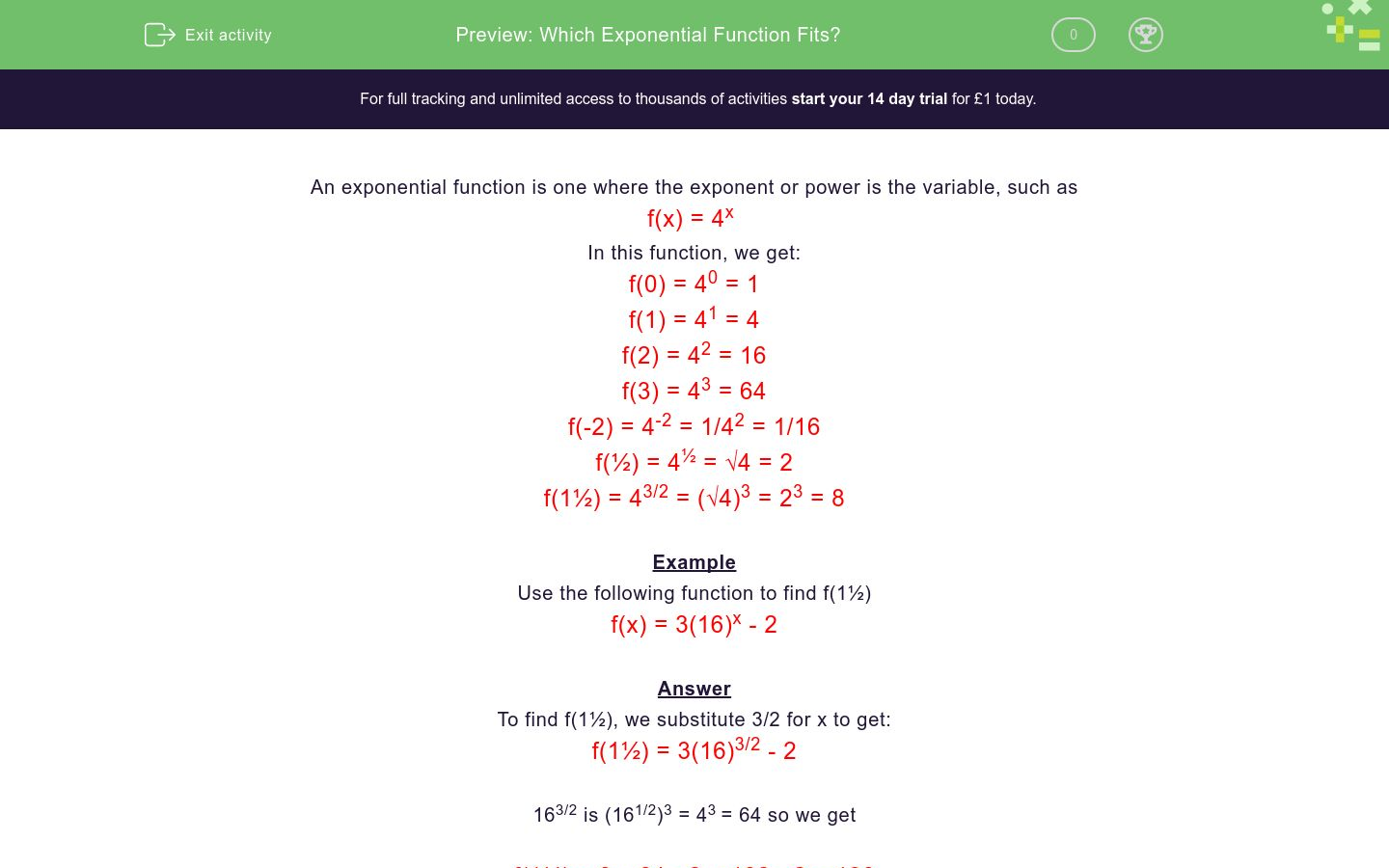# Which Exponential Function Fits?

In this worksheet, students choose the appropriate exponential function that fits the given data.Key stage:  KS 4

Curriculum topic:  Algebra

Difficulty level:### QUESTION 1 of 10

An exponential function is one where the exponent or power is the variable, such as

f(x) = 4x

In this function, we get:

f(0) = 40 = 1

f(1) = 41 = 4

f(2) = 42 = 16

f(3) = 43 = 64

f(-2) = 4-2 = 1/42 = 1/16

f(½) = 4½ = √4 = 2

f(1½) = 43/2 = (√4)3 = 23 = 8

Example

Use the following function to find f(1½)

f(x) = 3(16)x - 2

To find f(1½), we substitute 3/2 for x to get:

f(1½) = 3(16)3/2 - 2

163/2 is (161/2)3 = 43 = 64 so we get

f(1½) = 3 x 64 - 2 = 192 - 2 = 190

f(1½) = 190

Choose the function which fits the following results:

f(1) = 4

f(2) = 16

f(3) = 64

f(x) = 4x

f(x) = 2x

f(x) = 23x

Choose the function which fits the following results:

f(1) = 8

f(2) = 16

f(3) = 512

f(x) = 4x

f(x) = 2x

f(x) = 23x

Choose the function which fits the following results:

f(0) = 1

f(-1) = 0.125

f(2) = 64

f(x) = 4x

f(x) = 2x

f(x) = 23x

Choose the function which fits the following results:

f(0) = 1

f(-1) = 0.5

f(-2) = ¼

f(x) = 4x

f(x) = 2x

f(x) = 23x

Choose the function which fits the following results:

f(-1) = -1¾

f(0) = -1

f(1) = 2

f(x) = 4x - 2

f(x) = 16x + 4

f(x) = 24x - 4

Choose the function which fits the following results:

f(-1) = 4.0625

f(0) = 5

f(1) = 20

f(x) = 4x - 2

f(x) = 16x + 4

f(x) = 24x - 4

Choose the function which fits the following results:

f(-1) = -3.9375

f(0) = -3

f(1) = 12

f(x) = 4x - 2

f(x) = 16x + 4

f(x) = 24x - 4

Choose the function which fits the following results:

f(½) = 8

f(1) = 20

f(2) = 260

f(x) = 4x - 2

f(x) = 16x + 4

f(x) = 24x - 4

Choose the function which fits the following results:

f(½) = 0

f(3/2) = 120

f(2) = 620

f(x) = 5x - 25

f(x) = 5x + 5

f(x) = 25x - 5

Choose the function which fits the following results:

f(-1) = -24.8

f(3/2) = (√5)3 - 25

f(2) = 0

f(x) = 5x - 25

f(x) = 5x + 5

f(x) = 25x - 5

• Question 1

Choose the function which fits the following results:

f(1) = 4

f(2) = 16

f(3) = 64

f(x) = 4x
EDDIE SAYS
41 = 4
42 = 16
43 = 64
• Question 2

Choose the function which fits the following results:

f(1) = 8

f(2) = 16

f(3) = 512

f(x) = 23x
EDDIE SAYS
23 = 8
26 = 64
29 = 512
• Question 3

Choose the function which fits the following results:

f(0) = 1

f(-1) = 0.125

f(2) = 64

f(x) = 23x
EDDIE SAYS
20 = 1
2-3 = 1/8
26 = 64
• Question 4

Choose the function which fits the following results:

f(0) = 1

f(-1) = 0.5

f(-2) = ¼

f(x) = 2x
EDDIE SAYS
20 = 1
2-1 = ½
2-2 = ¼
• Question 5

Choose the function which fits the following results:

f(-1) = -1¾

f(0) = -1

f(1) = 2

f(x) = 4x - 2
EDDIE SAYS
4-1 - 2 = 0.25 - 2
40 - 2 = 1 - 2
41 - 2 = 4 - 2
• Question 6

Choose the function which fits the following results:

f(-1) = 4.0625

f(0) = 5

f(1) = 20

f(x) = 16x + 4
EDDIE SAYS
16-1 + 4 = 1 ÷ 16 + 4
160 + 4 = 1 + 4
161 + 4 = 16 + 4
• Question 7

Choose the function which fits the following results:

f(-1) = -3.9375

f(0) = -3

f(1) = 12

f(x) = 24x - 4
EDDIE SAYS
2-4 - 4 = 1 ÷ 16 - 4
20 - 4 = 1 - 4
24 - 4 = 16 - 4
• Question 8

Choose the function which fits the following results:

f(½) = 8

f(1) = 20

f(2) = 260

f(x) = 16x + 4
EDDIE SAYS
16½ + 4 = √16 + 4
161 + 4 = 16 + 4
162 + 4 = 256 + 4
• Question 9

Choose the function which fits the following results:

f(½) = 0

f(3/2) = 120

f(2) = 620

f(x) = 25x - 5
EDDIE SAYS
25½ - 5 = √25 - 5
253/2 - 5 = (√25)³ - 5
252 - 5 = 625 - 5
• Question 10

Choose the function which fits the following results:

f(-1) = -24.8

f(3/2) = (√5)3 - 25

f(2) = 0

f(x) = 5x - 25
EDDIE SAYS
5-1 - 25 = 0.2 - 25
53/2 - 25 = (√5)³ - 25
52 - 25 = 25 - 25
---- OR ----

Sign up for a £1 trial so you can track and measure your child's progress on this activity.

### What is EdPlace?

We're your National Curriculum aligned online education content provider helping each child succeed in English, maths and science from year 1 to GCSE. With an EdPlace account you’ll be able to track and measure progress, helping each child achieve their best. We build confidence and attainment by personalising each child’s learning at a level that suits them.